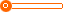# 豪华带电动百叶推拉门高端铝合金门窗厂家

1 平方米

10000 平方米

(发货期限：自买家付款之日起 天内发货)

• VIP指数:[第1年]
• 认证信息:
• 所在地区：广东
•• 详细说明
• 规格参数
• 联系方式

r ST-130重型推拉门产品特征： r

r

r 采用德国G-U（格屋）,HOPO（好博）,SIEGENIA(丝吉利娅）等高档品牌五金配件,单扇承重400KG，确保门扇推拉顺畅,平顺,无噪音。r

r

r 宽敞的采景玻璃,推拉灵活的落地推拉扇，使室外景色一览无余，尽收眼底。r

r

r 可选用提升配件,通过转动执手,门扇可随处固定,开启宽度随心所欲。r

r

r 推拉框通过EPDM密封胶条密封,彻底解决了传统推拉框扇结合部位采用毛条密封不严的难题。r

r

r 标配5mm+27A+5mm中空玻璃，可内置手动或者智能百叶系统。r

r

r 高科技的型材设计确保隔热,隔音和安全等性能。r

r

r
r

r

r 铝合金色卡： r

r

r
r

r

r
r
r

r

r 锁具： r

r

r
r
r r

r

r
r
r
r
r玻璃工艺： r

r

r
r
r包装：
r
r
r
r r

r

r 材料库存：
r
r r

r

r
r

r

r
r

r

r 出口装柜： r

r

r
r
r
r

"};

查看更多同类招商加盟产品
上一组 下一组

## 您可以通过以下类目找到类似信息：

免责声明：以上所展示的信息由会员自行提供，内容的真实性、准确性和合法性由发布会员负责。全球风时尚网对此不承担任何责任。

友情提醒：为规避购买风险，建议您在购买相关产品前务必确认供应商资质及产品质量！